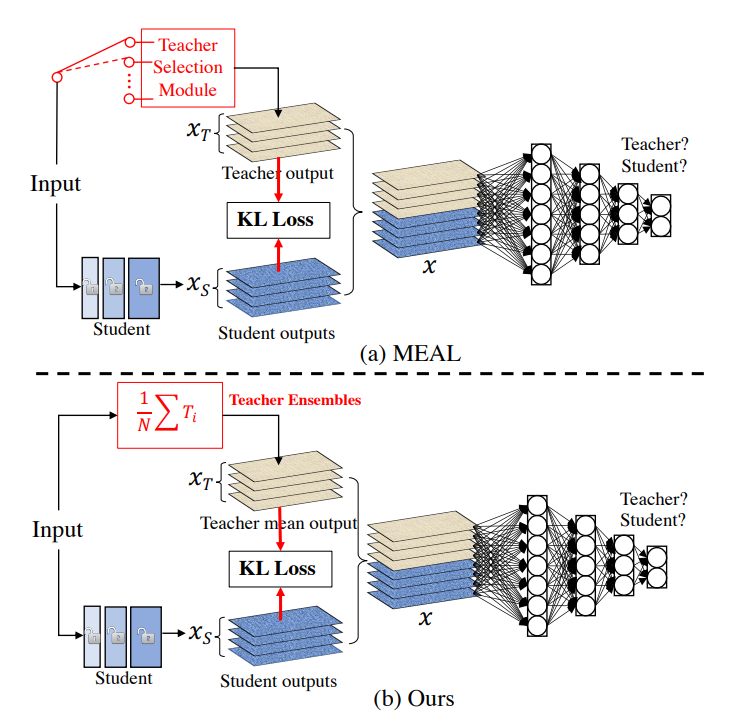MEAL V2主要的思路是将多个模型的集成效果通过知识蒸馏迁移到一个单一网络中，整个设计非常简单，只包括三个重要的部分：teacher模型集成，KL散度loss以及一个判别器。相比其它方法，不需要特殊的trick：

MEAL V2是MEAL方法的升级版，相比之下V2版本设计上更简单，效果也更好：

### Teacher模型集成

${\stackrel{^}{p}}_{e}^{{T}_{\theta }}=\frac{1}{K}\sum _{t=1}^{K}{p}_{t}^{{T}_{\theta }}\left(X\right)\phantom{\rule{0ex}{0ex}}$

### KL散度

KL散度可以用来衡量两个概率分布的差异，在训练过程中通过最小化student的概率输出${p}^{{S}_{\theta }}\left({x}_{i}\right)$和teacher模型集成后概率${\stackrel{^}{p}}^{{T}_{\theta }}\left({x}_{i}\right)$的KL散度来完成知识蒸馏。这里的损失函数如下：

$\begin{array}{rl}{L}_{KL}\left({S}_{\theta }\right)& =-\frac{1}{N}\sum _{i=1}^{N}{\stackrel{^}{p}}^{{T}_{\theta }}\left({x}_{i}\right)\mathrm{log}\left(\frac{{p}^{{S}_{\theta }}\left({x}_{i}\right)}{{\stackrel{^}{p}}^{{T}_{\theta }}\left({x}_{i}\right)}\right)\\ & =-\frac{1}{N}\sum _{i=1}^{N}{\stackrel{^}{p}}^{{T}_{\theta }}\left({x}_{i}\right)\mathrm{log}\left({p}^{{S}_{\theta }}\left({x}_{i}\right)\right)+\frac{1}{N}\sum _{i=1}^{N}{\stackrel{^}{p}}^{{T}_{\theta }}\left({x}_{i}\right)\mathrm{log}\left({\stackrel{^}{p}}^{{T}_{\theta }}\left({x}_{i}\right)\right)\end{array}\phantom{\rule{0ex}{0ex}}$

${L}_{CE}\left({S}_{\theta }\right)==-\frac{1}{N}\sum _{i=1}^{N}{\stackrel{^}{p}}^{{T}_{\theta }}\left({x}_{i}\right)\mathrm{log}\left({p}^{{S}_{\theta }}\left({x}_{i}\right)\right)\phantom{\rule{0ex}{0ex}}$

### 判别器

MEAL V2采用对抗学习来防止student在训练数据上过拟合，即不让student过分学习teacher的输出，这其实是一种正则化手段。具体做法是加入一个判别器来区分student的输出和teacher的输出，这是一个二分器。二分器采用一个3层FC的子网络，其输入是softmax前的logits。这里的student网络其实充当了生成器的角色，与传统的GAN训练方式不同，这里直接把判别器loss和前面所述的CE loss直接加起来一起训练，具体做法是每个batch中，teacher的输出其GT是[0, 1]，而student的输出其GT是[1,0]：

class discriminatorLoss(nn.Module):
def __init__(self, models, loss=nn.BCEWithLogitsLoss()):
super(discriminatorLoss, self).__init__()
self.models = models # 3层FC网络
self.loss = loss

def forward(self, outputs, targets):
"""
outputs和targets分别是student和teacher的logits
"""
inputs = [torch.cat((i,j),0) for i, j in zip(outputs, targets)]
inputs = torch.cat(inputs, 1)
batch_size = inputs.size(0)
target = torch.FloatTensor([[1, 0] for _ in range(batch_size//2)] + [[0, 1] for _ in range(batch_size//2)])
target = target.to(inputs.device)
output = self.models(inputs)
res = self.loss(output, target)
return res